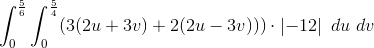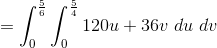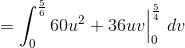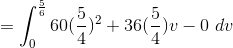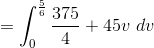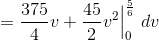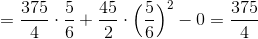# Calculus 3 : Double Integration with Change of Variables

## Example Questions

### Example Question #1 : Double Integration With Change Of Variables

Evaluate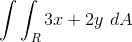, whereis the trapezoidal region with vertices given by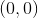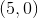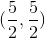, and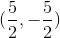,

using the transformation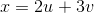, and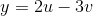.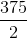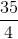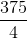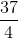Explanation:

The first thing we have to do is figure out the general equations for the lines that create the trapezoid.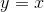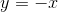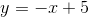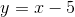Now we have the general equations for out trapezoid, now we need to plug in our transformations into these equations.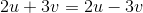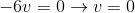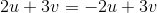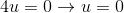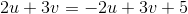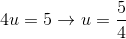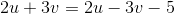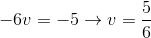So our region is a rectangle given by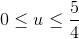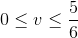Next we need to calculate the Jacobian.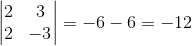Now we can put the integral together.